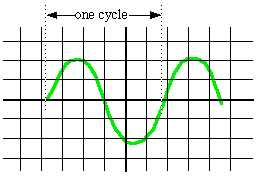# Hands-On-Physics WAVES

## - Frequency -

Test the Tone Generator's frequency.
You can attach the output of the tone generator directly to an oscilloscope. The horizontal line on the 'scope is actually a dot moving so rapidly it draws a line. The speed of the dot moving across the screen is controlled by the "horizontal sweep" knob. The sweep rate is usually given in seconds per division. In that case, the frequency is the number of scale divisions included in one cycle divided by the sweep rate (sec/div).Figure C4
Oscilloscope Measurement

In the picture above, one cycle includes about 5.7 divisions (0.9 + 4 + 0.8). If the sweep frequency were set at 0.02 second per division, the frequency of the wave pictured would be (5.7 / 0.02 ) = 285 Hz.

Determine the frequency produced by your Tone Generator.

Record the sweep rate and measure the length of one cycle, then calculate the frequency.

Previous Page || Up a Level || Index || Next Page